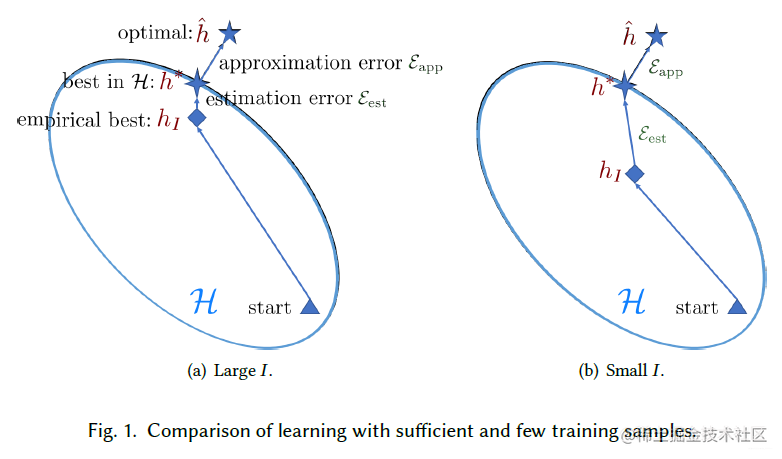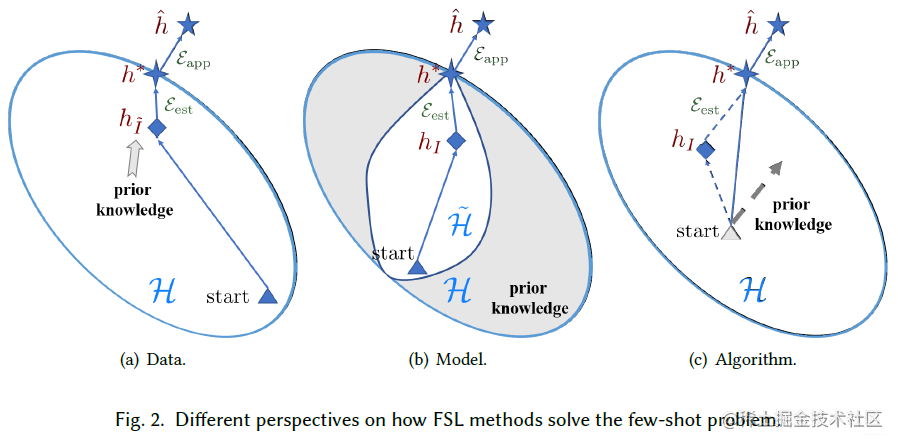# 【ACM2020】少样本学习综述

## 核心问题

机器学习中很大一部分的核心问题都在于误差分析，少样本也不例外，最核心的东西就是对误差的分析。按照机器学习的方式将few shot learning中的误差进行分解，然后进行分析。

给定假设hypothesis $h$，机器学习要做的事情就是最小化期望风险(expected risk) $R$

$R(h)=\int \ell(h(x), y) d p(x, y)=\mathbb{E}[\ell(h(x), y)]$

但是$p(x,y)$并不知道，因此往往采用从training set $D_{train}$sample一些样本来计算经验风险(empirical risk)$R_{I}$

$R_{I}(h)=\frac{1}{I}\sum_{i=1}^{I}\ell(h(x_{i}),y_{i})$

然后通过empirical risk 最小化来找到$h$。针对不同的假设有：

• $\hat{h}=arg\min_{h}R(h)$ 是最小化期望风险函数。
• $h^{*}=arg\min_{h \in \mathcal{H}}R(h)$是在$\mathcal{H}$下的期望风险函数，或者说$h^{*}$$\hat{h}$$\mathcal{H}$下的最好近似。
• $h_{I}=arg\min_{h\in \mathcal{H}}R_{I}(h)$是在$\mathcal{H}$下的经验风险函数。

整个误差就可以表示为：

$\mathbb{E}\left[R\left(h_{I}\right)-R(\hat{h})\right]=\underbrace{\mathbb{E}\left[R\left(h^{*}\right)-R(\hat{h})\right]}_{\mathcal{E}_{\mathrm{app}}(\mathcal{H})}+\underbrace{\mathbb{E}\left[R\left(h_{I}\right)-R\left(h^{*}\right)\right]}_{\mathcal{E}_{\mathrm{est}}(\mathcal{H}, I)}$

$\mathcal{E}_{\mathrm{est}}(\mathcal{H}, I)$可以通过sample大量的数据来减小，但是在few shot learning中数据很少，这一项的误差会比较大：## 少样本学习的解决办法

因为empirical risk minimizer $h_{I}$已经不可信了，因此必须引入先验知识(prior knowledge)来降低这个误差，大体上可以分为三个部分，从数据角度。从模型角度、从算法角度做改进：• Data：对训练数据$D_{train}$做数据增强，增加训练样本数量，将样本数量从$I$变到$\tilde{I}$，其中$\tilde{I} \gg I$，因此就能获得在$\tilde{I}$下的empirical risk minimizer $h_{\tilde{I}}$self-supervise learning也属于这一类。也有提出AutoAugment 4算法。这种方法一般用于图像，而对于文本，语音等方面没有太多应用。

J. Wei and K. Zou. 2019. EDA: Easy data augmentation techniques for boosting performance on text classification tasks. In Conference on Empirical Methods in Natural Language Processing and International Joint Conference on Natural Language Processing. 6383–6389.(思考：能否用于trajector的数据增强？)

• Model：利用先验知识约束$\mathcal{H}$的复杂性，从而得到一个更易优化的假设$\tilde{\mathcal{H}}$，使得在$\tilde{\mathcal{H}}$下小样本$D_{train}$能够获得一个可信的$h_{I}$1,2,3，迁移学习，预训练，Multitask learning就是这种方法。

• Algorithm：这种方式就是元学习的方法，通过先验知识提供一个初始化参数(上图c中的灰色三角形)，或者提供一个梯度搜索方向(上图c中的灰色虚线箭头)。

## 参考

• Generalizing from a Few Examples: A Survey on Few-Shot Learning